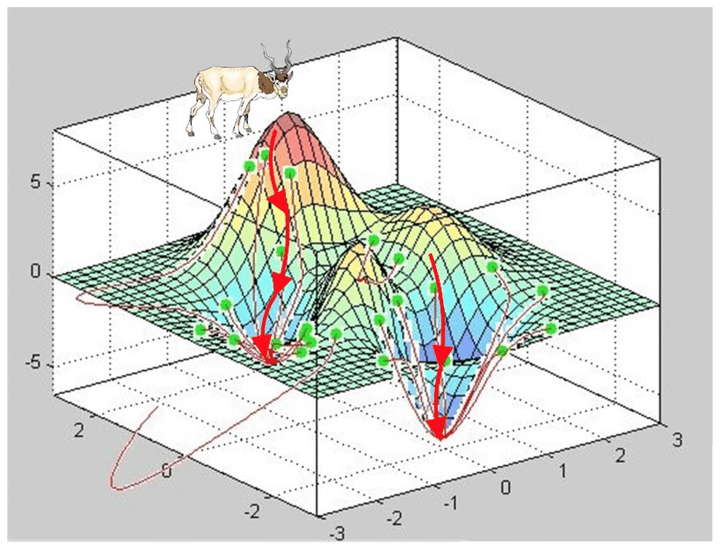# How to understand gradient descent?Gradient descent is a first-order iterative optimization algorithm for finding a local minimum of a differentiable function. To find a local minimum of a function using gradient descent, we take steps proportional to the negative of the gradient (or approximate gradient) of the function at the current point. But if we instead take steps proportional to the positive of the gradient, we approach a local maximum of that function; the procedure is then known as gradient ascent. Gradient descent is generally attributed to Cauchy, who first suggested it in 1847, but its convergence properties for non-linear optimization problems were first studied by Haskell Curry in 1944.